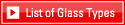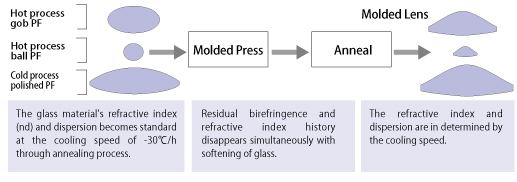Top > Products > Preforms for precision moldings > Refractive Index of PF

##Residual birefringence disappears at the viscosity in which glass is softened and pressed. The final refractive index is determined by the cooling speed through annealing.In general the faster the cooling speed, the lower the refractive index.When PF is annealed at the cooling speed of -30°C/h from the glass softening temperature, the standards or tolerances of refractive index of PF is guaranteed. The refractive index also can be impacted by the annealing conditions. Simple annealing is applied to PF we supply. It does not mean that the refractive index PF listed in the catalogue is guaranteed within specification. It is necessary to anneal PF at given cooling speed in order to acquire refractive index and dispersion within the specification.The refractive index change to the cooling speed varies slightly with light wavelength.The refractive index change (β values) is shown at d line, C line, F line and g line respectively. Thus, you can estimate the dispersion change according to the cooling speed change.

The refractive index change to the cooling velocity is expressed with the value of 10-5, the refractive index difference between the cooling velocity of -1°C/h and cooling velocity of -10°C/h with the unit of 10-5 and βx is measured at g line, F line, d line, and C line.

β = n R1 - n R10

Generally, logarithm value (logR) of cooling velocity and refractive index is a straight line relationship. The refractive index at d line for example, is shown in the following equation.

nd Rx = nd R1 - βlog(Rx)

Where nd Rx is nd on of the cooling velocity R (degree C/h).
nd R1 is nd on of the cooling velocity 1 (degree C/h).